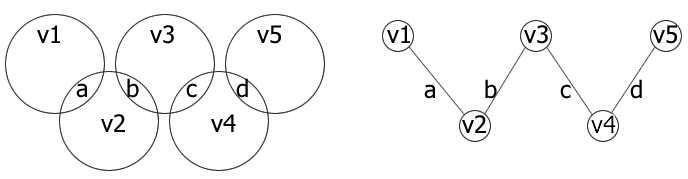#### You may also like### Instant Insanity

Given the nets of 4 cubes with the faces coloured in 4 colours, build a tower so that on each vertical wall no colour is repeated, that is all 4 colours appear.### Network Trees

Explore some of the different types of network, and prove a result about network trees.### Magic Caterpillars

Label the joints and legs of these graph theory caterpillars so that the vertex sums are all equal.

# Olympic Magic

##### Age 14 to 16Challenge Level

Thank you to Alma Iulia Dimitriu, of St Peter's RC High School, and member of the NRICH/NAGTY mentoring scheme), for this solution.We are working here with vertex magic graphs.

When we draw a graph for the problem there are 5 vertices and 4 edges. A vertex sum is the sum of the numbers on the vertex and on the edges joined to that vertex. The sum in each circle (which can be either 10,11,12,13,14 or15) is the vertex sum in the graph and we want to make this a constant X to make it a magic sum.

The sum of all the numbers is: 1+2+3+4+5+6+7+8+9 = 45

The edges are used twice. There are 4 numbers included in two circles and the sum of the 4 edges that are used twice is a+b+c+d=n, so n + 45 = 5X. So n has to be multiple of 5.

Also 1+2+3+4 = 10 so X must be greater than or equal to 10. So which means that 10 cannot be the magic sum.

For X to be 15, n has to be 30 and the only 4 numbers that add up to 30 are 6+7+8+9. It is impossible for any of them to be on the edges because the only pairs of numbers that add up to 15 are 9+6 and 7+8 and two of these numbers have to be placed at the two outer vertices.

For X to be 12, n has to be 15. There can be 3 pairs of numbers that equal 15, that is 10+5; 9+6 and 8+7. There are 4 numbers to make n because there are 4 edges. So all the possible choices of the 4 numbers on the edges are:

 For 10+5 For 9+6 For 8+7 1+9+2+3 1+8+2+4 1+7+2+5 2+8+4+1 2+7+1+5 1+7+3+4 3+7+4+1 3+6+2+4 2+6+3+4 4+6+2+3 3+6+1+5 3+5+1+6

The only two numbers that equal 12 (for the 2 circles on the margins) are: 9+3; 8+4 and 7+5.

So v1 and v5 have to be any two of those numbers. So now the possibilities left for the edges are:

For 10+5:

1+9+2+3 - no solution as the only two numbers here are 3+9=12 (they can't be separated)
2+8+4+1 - no solution the same as above
3+7+4+1 - a possible solution because for v1=7 and v5=4
4+6+2+3 - no solution

For 9+6: - no solutions

For 8+7: 1+7+3+4 (the same as the one above)

So now we only have two possible solutions where 7 and 4 have to be on the margins:

Case (i) a=4, b=1, c=3, d=7
a=4 needs v1=8 to make 12. The second circle already has 4 and 1 so it will need v2=7 to make 12. This cannot be a solution because we already have d=7.

Case (ii) a=7, b=1, c=3, d=4
a=7 will need v1=5 to make 12. The second circle has 7 and 1 and it will need v2=4 but this is impossible as d=4.

So there is no possible solution with X equal to10, 12 or 15

The remaining values for X are 11, 13 and 14 for which 5X=55, 65 and 70. As n + 45 = 5X we have n=10, 20 and 25.

For X=11, the only numbers for the edges to add up to 10 are: 1+2+3+4 and the only pairs to make 11 are: 9+2, 8+3, 7+4 and 6+5. Checking all the possible cases gives the following solution and its mirror image:

v1=8, a=3, v2=7, b=1, v3=6, c=4, v4=5, d=2, v5=9

For X=13 we have a+b+c+d=n=20 and checking all possible cases gives two solutions and their mirror images;

v1=9, a=4, v2=1, b=8, v3=3, c=2, v4=5, d=6, v5=7
v1=4, a=9, v2=1, b=3, v3=8, c=2, v4=5, d=6, v5=7

For X=14 we have a+b+c+d=n=25 and one solution with its mirror image

v1=5, a=9, v2=2, b=3, v3=4, c=7, v4=1, d=6, v5=8